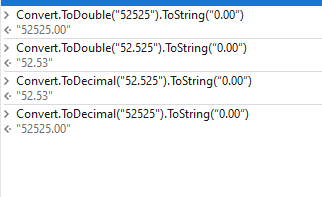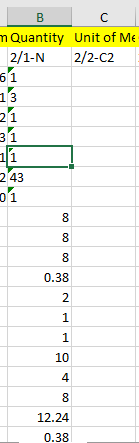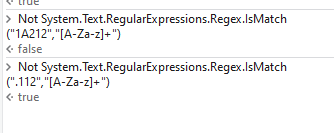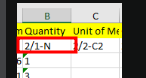# String To integer1

Hey I have one row which have no as string I want all no as integer format Like(0.00)
Can we have any code or any solution for this

Use `Convert.ToDouble` method
If you want the format “0.00” then use
outputString = Convert.ToDouble(inputString).ToString(“0.00”)

Try this

Int=Cdbl(String)

@Kuldeep_Pandey

For Each Row in dt_ProcessName
{
Assign - row(“ColumnName”) = String.Format(“{0:0.00}”, Convert.ToDouble(row(“ColumnName”).ToString()))
}

Can you try with the following expression

``````Convert.ToDecimal("52525").ToString(“0.00”)
``````Can you share the sample input?

Regards
Gokul

Hi @Kuldeep_Pandey ,
strOutput = Convert.ToDouble(strInput).ToString(“0.00”)
Regards,Sample Input I want to change quantity format as integer

Test.xlsx (11.0 KB)
I want to change quantity type

Hi @Kuldeep_Pandey,
Whenever you’ll try to write the data back to excel as 0.00 it’ll revert back to 0 or 14.00 to 14

If you need it to look like with decimal points.

Use Excel activities: Format Cells and set format as Number with 2 Decimals.

That would do what’s needed.

Also provide the range.

Happy Automation!

Use if activity to manuplate the alpabet in the quantity column

``````Condition -> Not System.Text.RegularExpressions.Regex.IsMatch(".112","[A-Za-z]+")
``````

Then - > Use Assign activity

``````Convert.ToDecimal("52525").ToString(“0.00”)
``````Regards
Gokul

We dont have any alphabet in quantity column

Then what is this from the ScreenshotRegards
Gokul

I don’t Know the range